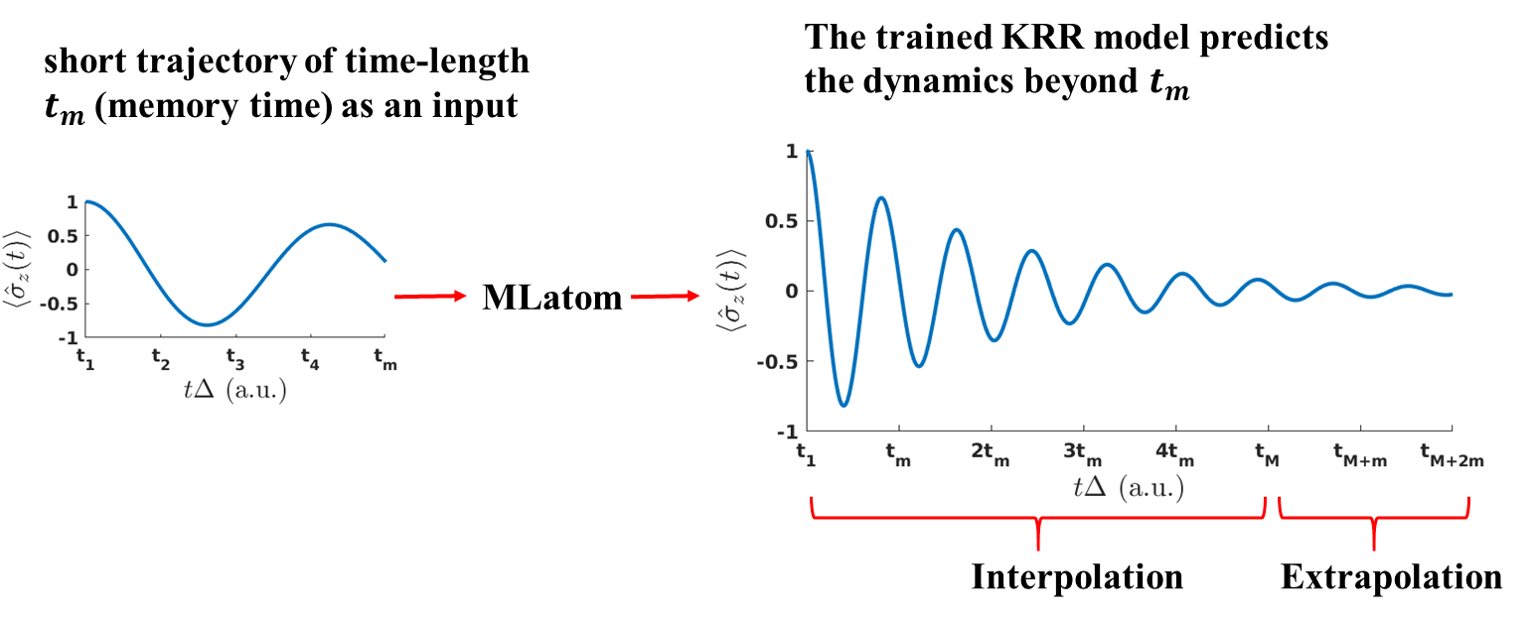# Speeding up quantum dissipative dynamics of open systems with kernel methodsIn the work published in New Journal of Physics, we combine machine learning (ML) with the numerically exact hierarchical equations of motion (HEOM) approach, propagating quantum dynamics of a two-state system (spin-boson model) with only ca. 10% of the HEOM computational cost. Our approach is not limited to the HEOM method and can be combined with any numerically exact method.

In the field of quantum mechanics, we aim for exact methods but they all come with a high computational cost. Because of the curse of dimensionality, exact simulations are limited to systems with only a few degrees of freedom and for comparatively large systems we always need to use some approximations. Coming to the field of dynamics, as no system in real world is isolated, including environmental effects is crucial. Many exact methods such as HEOM, QuAPI and SEOM are developed, promising the exact dynamics of the system under the influence of the environment. However, because of the exponential increase in dimensionality, these methods are very expensive.

To decrease the computational of these methods, ML is a promising tool. In our study (open access), we show that by providing a short-time trajectory as an input, a trained ML model can predict the beyond dynamics in a few seconds, decreasing the computational cost by ca. 90 %. In addition, in contrast to traditional approaches where computational cost is usually dependent on temperature, reorganization energy, and other parameters, the computational cost of ML remains the same for all cases. Moreover, our ML model is able to accurately extrapolate beyond the time region it was trained on, i.e., the training trajectories were much shorter than the test trajectories. Calculations were performed with our publicly available MLatom package utilizing the kernel ridge regression algorithm with the Gaussian kernel function. The simplicity and speed of training this algorithm make it a good alternative to other ML approaches for learning time-series such as convolutional neural networks (CNN) and recurrent neural networks which were suggested by other authors in related works on accelerating quantum dynamics. In fact, the previous work on CNN by Herrera Rodriguez and Kananenka was an inspiration for our work. In contrast to these related works, our model also directly learns the property of interest (population difference) rather than intermediate properties.

Our method can be applied to the low-cost propagation of long-time dynamics to alleviate the limitations of traditional methods. For example, our approach can be used to accelerate SEOM-level dynamics, where good convergence in the long-time regime needs a large number of trajectories, making the method expensive. ML will be very useful to accelerate HEOM method (as we have shown in our study) which is very expensive at low temperatures and hence long-time propagation can be quite challenging.

The data, code, and instructions can be found on GitHub, see the second case study (part of our upcoming chapter on Excited-state dynamics with machine learning).

### 0 Comments on “Speeding up quantum dissipative dynamics of open systems with kernel methods”

This site uses Akismet to reduce spam. Learn how your comment data is processed.